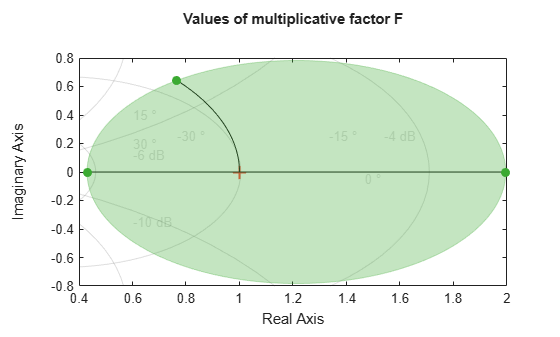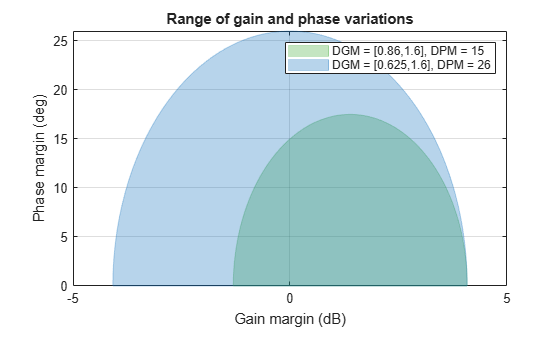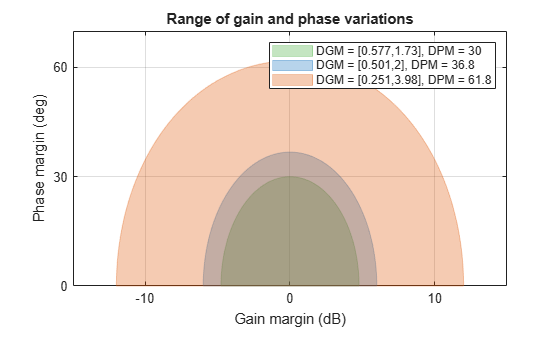# getDGM

Convert gain and phase variation into disk-based gain variation

## Syntax

``DGM = getDGM(GM,PM,'tight')``
``DGM = getDGM(GM,PM,'balanced')``
``[DGM,DPM] = getDGM(___)``

## Description

In disk margin analysis, gain and phase variations are modeled as a factor F(s) multiplying the open loop response L(s). This factor takes values in a disk D centered on the real axis with real-axis intercepts `gmin` and `gmax`. The disk margin determines the largest disk size `[gmin,gmax]` for which the feedback loop remains stable. This provides a gain margin of at least `DGM = [gmin,gmax]` and also some phase margin `DPM` determined by the disk geometry.Conversely, `getDGM` takes desired gain and phase margins `GM` and `PM` and computes the smallest disk D that delivers both. This disk is characterized by its real-axis intercepts `gmin`, `gmax` and the corresponding disk-based gain margin `DGM = [gmin,gmax]` and phase margin `DPM` meet or exceed `GM` and `PM`.

example

````DGM = getDGM(GM,PM,'tight')` computes the smallest disk that captures the target gain and phase variations specified by `GM` and `PM`. If `GM` and `PM` are scalars, then the disk captures gain that can increase or decrease by a factor of `GM`, and phase that can increase or decrease by `PM`. If `GM` and `PM` are vectors of the form `[glo,ghi]` and `[pmin,pmax]` then the disk captures relative gain and phase variations in these ranges.If either `GM` or `PM` is `[]`, that removes the corresponding constraint on the disk size.The output is of the form `DGM` = `[gmin,gmax]`, and describes a disk that represents absolute gain variations within that range. For instance, `DGM = [0.8,1.8]` models gain that can vary from 0.8 times the nominal value to 1.8 times the nominal value, and phase variations determined by the disk geometry. This disk might have non-zero skew (see Algorithms). Use `DGM` to create a `umargin` block that models these gain and phase variations.```

example

````DGM = getDGM(GM,PM,'balanced')` computes the smallest disk that represents a symmetric gain variation, that is, `DGM` = `[gmin,gmax]` where `gmin` = `1/gmax`. This disk has zero skew (see Algorithms). ```

example

````[DGM,DPM] = getDGM(___)` also returns the disk-based phase range `DPM` modeled by the disk that `DGM` describes. You can use this output argument with any of the previous syntaxes.```

## Examples

collapse all

Find the smallest disk-based gain margin that represents relative a gain variation of ±6 dB relative to the nominal value and phase variation of ±40°. Convert the gain variation into absolute units.

`GM = db2mag(6)`
```GM = 1.9953 ```
```PM = 40; DGM = getDGM(GM,PM,'tight')```
```DGM = 1×2 0.4299 1.9953 ```

`DGM` describes a disk that models both gain and phase variations. The values in `DGM` represent the range of gain variation in the absence of phase variation. Note that the `DGM` range is slightly larger than the specified `[1/GM,GM]` range as the phase margin requirement is more stringent and determines the disk size. Visualize the full range of gain and phase variations represented by `DGM`.

`diskmarginplot(DGM)`The '`tight`' constraint computes the smallest disk that delivers both target gain and phase variations, which does not necessarily represent a symmetric gain range. In this case, the disk represents gain that can decrease somewhat more than it can increase. Examine the disk of uncertainty defined by this particular `DGM`.

`diskmarginplot(DGM,'disk')`To enforce symmetric gain variation, use the `'balanced'` option.

Determine the disk-based gain margin that delivers symmetric gain variation of $±$5 dB and phase variation of $±$30 degrees.

```GM = db2mag(5); PM = 30; DGM = getDGM(GM,PM,'balanced')```
```DGM = 1×2 0.5623 1.7783 ```

The '`balanced`' constraint models a disk of uncertainty that is symmetric around the nominal value. The function returns a symmetric disk-based gain margin `DGM` = `[gmin,gmax]`, with `gmin=1/gmax`.

`diskmarginplot(DGM)`In this case, `DPM` slightly exceeds the target phase variation and `DGM` is equal to the target gain variation.

Determine the disk-based gain margin corresponding to gain variations between 90% and 160% of the nominal value, and phase variations from -15 to +15 degrees.

```gainRange = [0.9,1.6]; phaseRange = [-15,15]; DGMt = getDGM(gainRange,phaseRange,'tight')```
```DGMt = 1×2 0.8603 1.6000 ```

The '`tight`' constraint models the smallest disk that delivers target gain and phase variations. This disk is modeled with gain variation that skews toward gain increase.

Alternatively, you can use the '`balanced`' option to constrain the disk-based gain margin to a symmetrical range of the form `gmin = 1/gmax`. This means that the gain can increase or decrease by equal amount.

`DGMb = getDGM(gainRange,phaseRange,'balanced')`
```DGMb = 1×2 0.6250 1.6000 ```

Visualize the range of simultaneous gain and phase variations corresponding to both gain ranges.

`diskmarginplot([DGMt;DGMb]) `The balanced range `DGMb` models a larger, symmetric gain range (`gmin = 1/gmax`) and larger phase variations than the ones you specify. If you are confident that gain varies more in one direction than the other in your system, then this balanced model might be overly conservative.

Determine the balanced disk-based gain margin ranges that delivers gain variations of ±4 dB, ±6 dB, and ±12 dB and phase variation of ±30°. You can get all the disk-based gain ranges at once by stacking the desired target ranges into a column vector.

```GM = db2mag([4;6;12]); PM = 30; DGM = getDGM(GM,PM,'balanced')```
```DGM = 3×2 0.5774 1.7321 0.5012 1.9953 0.2512 3.9811 ```
`diskmarginplot(DGM)`Each row in the matrix `DGM` gives the disk-based gain variation for the corresponding entry in `GM`. For instance, the smallest balanced (symmetric) disk that captures gain variation of ±4 dB and phase variation of ±30° is specified by `DGM(1,:) = [0.58 1.73]`.

This disk represents somewhat more than the target ±4 dB, in order to capture the full target gain variation of ±30°. For the targets ±6 dB and ±12 dB, the disk meets the target gain variation exactly, but the corresponding disks describe larger phase variations.

## Input Arguments

collapse all

Target range of relative gain variation, specified as a scalar, vector, or two-column matrix.

• If `GM` is a scalar, then the disk captures gain that can increase or decrease by a factor of `GM`. For instance, if `GM` = 2, then the output `DGM` represents gain that can decrease or increase by a factor of 2.

• If `GM` is a vectors of the form `[glo,ghi]` then the disk captures relative gain variations in this range. For instance, if `GM`` = [0.8,1.9]`, then `DGM` represents gain that can vary between 0.8 and 1.9 times the nominal value.

• If `GM` `[]`, then `getDGM` returns a disk that captures the phase variation specified by `PM`, and the corresponding gain variation determined by the disk model.

#### Multiple Ranges at Once

To get `DGM` corresponding to multiple target gain ranges at once, specify `GM` as a column vector `[GM1;...;GMn]` or a matrix `[glo1,ghi1;...;gloN,ghiN]`.

Target phase variation, specified as a scalar, vector, or two-column matrix.

• If `PM` is a scalar, then the disk captures phase that can increase or decrease by `PM`. For instance, if `PM` = 20, then the output `DGM` represents phase that can vary by ±20°.

• If `PM` is a vector of the form `[pmin,pmax]` with `pmin < 0` and `pmax > 0`, then the disk captures phase that can vary by ±`min(abs(pmin),pmax)`. For instance, if `[pmin,pmax]` = `[-20,40]` then the disk captures phase variation in the range `[-40,40]`.

• If `PM` `[]`, then `getDGM` returns a disk that captures the relative gain variation specified by `GM`, and the corresponding phase variation determined by the disk model.

#### Multiple Ranges at Once

To get `DGM` corresponding to multiple target phase ranges at once, specify `PM` as a column vector `[PM1;...;PMn]` or a matrix of the form `[-pm1,pm1;...;-pmN,pmN]`.

## Output Arguments

collapse all

Modeled range of relative gain variation, returned as a two-element vector of the form `[gmin,gmax]`, where `gmin` < 1 and `gmax` > 1. For instance, `DGM = [0.8 1.5]` represents a gain that can vary between 80% and 150% of its nominal value (that is, change by a factor between 0.8 and 1.5). `gmin` can be negative, defining a range of relative gain variation that includes a change in sign. When you use the `'balanced'` option, the gain change is symmetric, that is, the gain can increase or decrease by the same amount (`gmin` = `1/gmax`).

The range `[gmin,gmax]` describes a disk of gain and phase uncertainty where the gain can vary by `[gmin,gmax]` and the phase can vary by an amount determined by the disk geometry. For instance, the following plot shows a disk characterized by `DGM = [0.5,2]` (For more information about the disk-based uncertainty model, see Algorithms). The corresponding phase variation (returned in `DPM`) is ±30°.In general, `DGM` or the corresponding `DPM` might capture larger ranges of variation than those you specify with the inputs `GM` and `PM`. The disk always captures at least the specified variations.

If `GM` is a column vector or matrix representing multiple target ranges of gain variation, `DGM` is a two-column matrix of the form `[gmin1,gmax1; ...;gminN,gmaxN]`, where each row is a corresponding disk-based gain range.

Disk-based phase margin, returned as a two-element vector of the form `[-pm,pm]`. The amount of phase variation is determined by the geometry of the disk described by `DGM` (see Algorithms).

If `PM` is a column vector or matrix representing multiple target ranges of phase variation, `DPM` is a two-column matrix of the form `[-pm1,pm1; ...;-pmN,pmN]`, where each row is a corresponding disk-based gain range.

## Algorithms

`umargin` and `diskmargin` model gain and phase variations in an individual feedback channel as a frequency-dependent multiplicative factor F(s) multiplying the nominal open-loop response L(s), such that the perturbed response is L(s)F(s). The factor F(s) is parameterized by:

`$F\left(s\right)=\frac{1+\alpha \left[\left(1-\sigma \right)/2\right]\delta \left(s\right)}{1-\alpha \left[\left(1+\sigma \right)/2\right]\delta \left(s\right)}.$`

In this model,

• δ(s) is a gain-bounded dynamic uncertainty, normalized so that it always varies within the unit disk (||δ|| < 1).

• ɑ sets the amount of gain and phase variation modeled by F. For fixed σ, the parameter ɑ controls the size of the disk. For ɑ = 0, the multiplicative factor is 1, corresponding to the nominal L.

• σ, called the skew, biases the modeled uncertainty toward gain increase or gain decrease.

The factor F takes values in a disk centered on the real axis and containing the nominal value F = 1. The disk is characterized by its intercept `DGM = [gmin,gmax]` with the real axis. `gmin` < 1 and `gmin` > 1 are the minimum and maximum relative changes in gain modeled by F, at nominal phase. The phase uncertainty modeled by F is the range `DPM = [-pm,pm]` of phase values at the nominal gain (|F| = 1). For instance, in the following plot, the right side shows the disk F that intersects the real axis in the interval [0.71,1.4]. The left side shows that this disk models a gain variation of ±3 dB and a phase variation of ±19°.

```DGM = [0.71,1.4] F = umargin('F',DGM) plot(F)````getDGM` converts the target gain and phase variations that you want to model into the disk-based gain-variation range `DGM`. This range fully characterizes the disk F. The corresponding phase range `DPM` is thus determined by `DGM` and the disk model.

For further details about the uncertainty model for gain and phase variations, see Stability Analysis Using Disk Margins.

### Topics

Introduced in R2020a

## SupportGet trial now